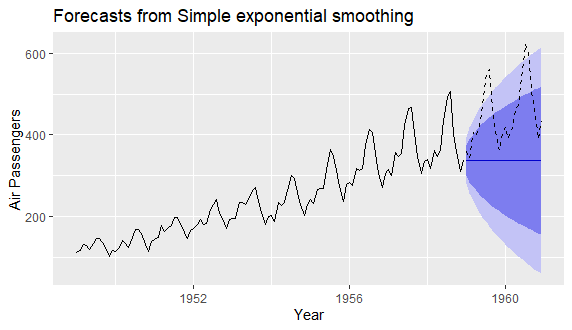# Exponential Smoothing: Brown Simple Method in R

Last Update: April 24, 2022

Exponential Smoothing: Brown Simple Method in R can be done using `forecast` package `ses` function for forecasting by flattening time series data with no trend or seasonal patterns. Main parameters within `ses` function are `y` with time series data, `h` with number of forecasting periods, `PI` with logical value on whether prediction intervals are estimated, `level` with prediction intervals statistical confidence levels and `initial` with initial state values estimation method.

As example, we can do model fitting and forecasting using Brown simple exponential smoothing method for airline passengers with training range as first ten years and testing range as last two years using data included within `datasets` package `AirPassengers` object .

First, we load packages `forecast` for Brown simple exponential smoothing method and `ggplot2` for its chart .

``````In :
library(forecast)
library(ggplot2)
``````

Second, we create `mdata` model data object copied from `datasets` package `AirPassengers` object and print first six months of data using `head` function to view time series object structure.

``````In :
mdata <- AirPassengers
``````
``````Out :
Jan Feb Mar Apr May Jun
1949 112 118 132 129 121 135
``````

Third, we delimit training range for model fitting as first ten years of data with `window` function and store outcome within `tdata` object. Within `window` function, parameters `x = mdata` includes full range model data and `end = c(1958, 12)` includes training range end time. Then, we delimit testing range for model forecasting as last two years of data with `window` function and store outcome within `fdata` object. Within `window` function, parameters `x = mdata` includes full range model data and `start = c(1959, 1)` includes training range start time. Notice that training and testing ranges delimiting was only included as an educational example which can be modified according to your needs.

``````In :
tdata <- window(x = mdata, end = c(1958, 12))
fdata <- window(x = mdata, start = c(1959, 1))
``````

Fourth, we fit and forecast Brown simple exponential smoothing method with `ses` function using `tdata` training range data object and store outcome within `brown` object. Within `ses` function, parameters `y = tdata` includes training range data, `h = 24` includes twenty four months or two years forecasting period, `PI = TRUE` includes logical value to estimate prediction intervals, `level = c(80, 95)` includes prediction intervals eighty and ninety five percent statistical confidence levels and `initial = "optimal"` includes optimized initial state values. Notice that initial state values can also be estimated using simple formula. Also, notice that `ses` function parameters were only included as educational examples which can be modified according to your needs. Additionally, notice it is important to remember that when doing time series analysis and forecasting, past performance does not guarantee future results.

``````In :
brown <- ses(y = tdata, h = 24, PI = TRUE, level = c(80, 95),
initial = "optimal")
``````

Fifth, we view Brown simple exponential smoothing method forecast with `autoplot`, `autolayer` and `labs` functions. Within `autoplot` function, parameter `object = brown` includes `brown` forecast object. Within `autolayer` function, parameters `object = fdata` includes testing range data object, `color = "black"` includes line color and `linetype = 2` includes dashed line type. Within `labs` function, parameters `y = "Air Passengers"` includes vertical axis label and `x = "Year"` includes horizontal axis label.

``````In :
autoplot(object = brown) +
autolayer(object = fdata, color = "black", linetype = 2) +
labs(y = "Air Passengers", x = "Year")
``````
``Out :``Figure 1. Model fitting and forecasting using Brown simple exponential smoothing method for airline passengers with training range as first ten years and testing range as last two years of data.

 Data Description: Monthly international airline passenger numbers in thousands from 1949 to 1960.

Original Source: Box, G. E. P., Jenkins, G. M. and Reinsel, G. C. (1976). “Time Series Analysis, Forecasting and Control”. Third Edition. Holden-Day. Series G.

Source: datasets R Package AirPassengers Object. R Core Team (2021). “R: A language and environment for statistical computing”. R Foundation for Statistical Computing, Vienna, Austria.

 forecast R Package. Hyndman R, Athanasopoulos G, Bergmeir C, Caceres G, Chhay L,
O’Hara-Wild M, Petropoulos F, Razbash S, Wang E, Yasmeen F (2022). “forecast: Forecasting functions for time series and linear models”. R package version 8.16

ggplot2 R Package. Hadley Wickham (2016). “ggplot2: Elegant Graphics for Data Analysis”. Springer-Verlag New York

+